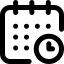# B.Sc. Applied Mathematics & Statistics

## DETAILS

B.Sc. Applied Mathematics & Statistics is the application of mathematics to real-world phenomena, both observed and yet to be observed. B.Sc. Applied Mathematics & Statistics will help our students to not only explain outcomes in nature and in human societies but also to predict them or, how to affect them. Applied Mathematics & Statistics training will help our students work alongside physical, biological and social scientists.

The student can choose between a 3-year Bachelor's degree or a 4-year Bachelor with Honours degree. For the 3-year degree, the student must complete a minimum of 120 credits through a combination of majors and minors. In the 4-year program, a student will have to complete 160 credits (120+40 credits), the additional credits made up of advanced courses and a project, or research. The 3-year program enables a student to join the Masters’ program while the 4-year program enables the student to directly enter the PhD program. Students can also opt for placement at the end of both, the 3-year program and the 4-year program.### Duration of Program

3 + 1 (optional) Years### Seats

20*

50% in 10th & 12th Math/Eco/Pol.Sc. in 12th will be preferred.
Non-Mathematics background students will undergo Remedial Mathematics module in Semester 1

Aptitude Test / Valid JEE Score / CUET 2022 score

 B.Sc. Applied Mathematics & Statistics Year Understanding Data 1 Real Analysis I (Calculus of one variable) 1 Probability Theory I 1 Algebra 1 Introduction to Programming and Data Structures (Python & R) 1 Real Analysis II (Metric spaces and Multivariate Calculus) 2 Probability Theory II 2 Linear Algebra 2 Introduction to Differential Equations 2 Real Analysis III (Vector Calculus) 2 Probability Theory III 2 Optimization Techniques 2 Numerical Analysis 2 Non-linear Optimization Techniques 3 Introduction to Stochastic Processes 3 Real Analysis IV (Introduction to Function Spaces) 3 Discrete Mathematics 3 Algebra III 3 Algebra IV 3 Complex Analysis 3 Introduction to Differential Geometry 3 Introduction to Algebraic Number Theory 3 Trigonometry and Analytical Geometry 3 Introduction to Representation Theory 3 Introduction to algebraic geometry 3 Differential Geometry II 3 Introduction to Differential Topology 3 Statistical Methods I 3 Linear Statistical Models 3 Parametric Inference 3 Sample Surveys 3 Economic and Official Statistics and Demography 3 Design and Analysis of Algorithms 3 Nonparametric and Sequential Methods 3 Design of Experiments 3 Statistical Quality Control and Operations Research 3 Statistics Comprehensive 3

*The year in which subjects are taught may change according to academic policies

Eligibility

50% in 10th & 12th Math/Eco/Pol.Sc. in 12th will be preferred.
Non-Mathematics background students will undergo Remedial Mathematics module in Semester 1

Non-Examination Pathway

Aptitude Test / Valid JEE Score / CUET 2022 score

Curriculum
 B.Sc. Applied Mathematics & Statistics Year Understanding Data 1 Real Analysis I (Calculus of one variable) 1 Probability Theory I 1 Algebra 1 Introduction to Programming and Data Structures (Python & R) 1 Real Analysis II (Metric spaces and Multivariate Calculus) 2 Probability Theory II 2 Linear Algebra 2 Introduction to Differential Equations 2 Real Analysis III (Vector Calculus) 2 Probability Theory III 2 Optimization Techniques 2 Numerical Analysis 2 Non-linear Optimization Techniques 3 Introduction to Stochastic Processes 3 Real Analysis IV (Introduction to Function Spaces) 3 Discrete Mathematics 3 Algebra III 3 Algebra IV 3 Complex Analysis 3 Introduction to Differential Geometry 3 Introduction to Algebraic Number Theory 3 Trigonometry and Analytical Geometry 3 Introduction to Representation Theory 3 Introduction to algebraic geometry 3 Differential Geometry II 3 Introduction to Differential Topology 3 Statistical Methods I 3 Linear Statistical Models 3 Parametric Inference 3 Sample Surveys 3 Economic and Official Statistics and Demography 3 Design and Analysis of Algorithms 3 Nonparametric and Sequential Methods 3 Design of Experiments 3 Statistical Quality Control and Operations Research 3 Statistics Comprehensive 3

*The year in which subjects are taught may change according to academic policies